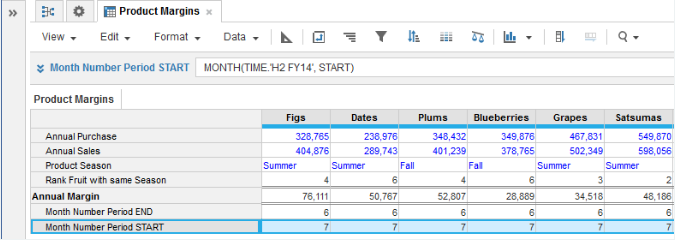1. Calculation functions
2. All Functions
3. Time and Date Functions
4. MONTHConverts a date or a time period to a month in number format.

The date format depends on your browser settings.

## Syntax

`MONTH(Value to convert[, Time period method])`
 Argument Data type Description Value to convert (required) Date, time period The date or time period to convert to a month as a number. Time period method (optional) Keyword Determines which date in a time period to extract the month from.

## Returns

The MONTH function returns a number-formatted result.

## Arguments

The Value to convert argument can refer to a line item, property, or expression. This means that you can also use explicit time references such as Time.‘Q2 FY20’ or Time.‘FY20’.

You can only use the Time period method argument if the Value to convert argument is time period-formatted or an explicit time reference. The keywords for the Time period method argument are:

START (default): returns the month for the first date in the time period

MID: returns the month for the day in the middle of the time period

END: returns the month for the last date in the time period

## Constraints

The MONTH function has these constraints:

• The Value to convert argument must be date- or time period-formatted.
• The result must be number-formatted.

## Examples

In this example, the source line item, Product Date, is date-formatted. The result line item, Product Month, is number-formatted. The function converts the dates to numbers that represent the relevant month.

`MONTH(Product Date) `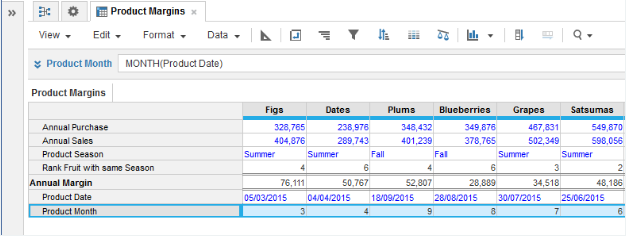In this example, the source line item, Month Period, is time period-formatted. The result line item, Month Number, is number-formatted. The function converts the time periods to numbers that represent the month.

`MONTH(Month Period)`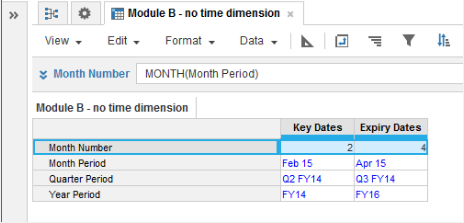This example uses an explicit time reference, TIME.'Apr 15', instead of a line item, for the Value to convert argument. The result line item, Month Number Abs, is number-formatted. The formula returns the month for TIME.'Apr 15' as a number.

```MONTH(TIME.'Apr 15')
```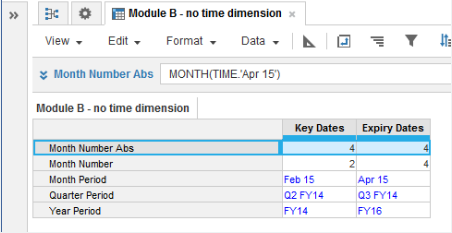In this example, the source line item, Product Quarter Period, is time period-formatted. The result line items are Product Month Qtr START, Product Month Qtr MID, and Product Month Qtr END. They use the START, MID, and END keywords for the Time period method argument. This returns the month as a number for the start, middle, and end dates of the quarter period.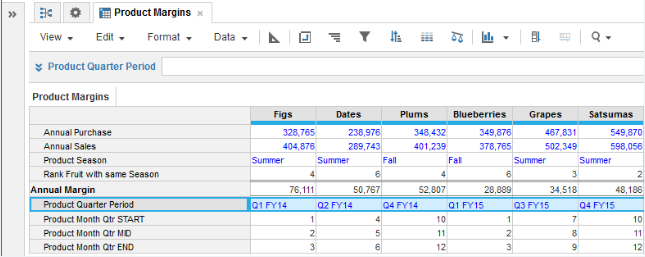This example uses an explicit time reference, TIME.'H1 FY14', instead of a line item, for the Value to convert argument. The Month Number Period END line item uses the END keyword for the Time period method argument. This returns the month as a number for the end date of the half-year period.

`MONTH(TIME.'H1 FY14', END)`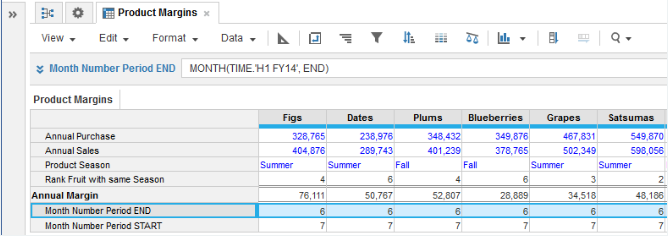This example uses an explicit time reference, TIME.'H2 FY14', instead of a line item, for the Value to convert argument. The Month Number Period START line item uses the START keyword for the Time period method argument. This returns the month as a number for the start date of the half-year period.

`MONTH(TIME.'H2 FY14', START)`